# Time and Distance

#### Video Lesson on Time and Distance

Time and Distance is one of the most important chapters for any entrance exam. The concepts of this chapter help you in dealing effectively with the other two chapters i.e “Trains” and “Boats and Streams”.

### Important Points to Remember

1. Speed is defined as the distance travelled per unit time.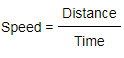2. If same distance X is travelled at two different speeds S1 & S2, then average speed is calculated by,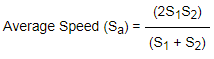3. Two bodies A and B move between two points P & Q. One starts from P and goes to Q while the other starts from Q and goes to P. They meet on the way and reach their destinations in time ta and tb respectively after meeting . Their speeds Sa & Sb are given by,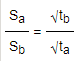4. When two bodies move in opposite direction, their speeds are added to find the relative speed. SR = S1 + S2
5. When two bodies move in same direction, their speeds are subtracted to find the relative speed. SR = S1 + S2### Conversion of Units

It is extremely important to pay attention to the units for each of the quantities given. At times, you may be required to convert the unit of speed from km per hr to metre per second or vice versa.

#### Converting km/hr into m/s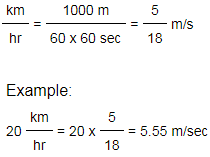#### Converting m/s into km/hr: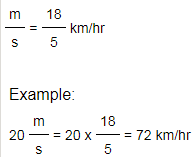• To convert minutes into seconds, multiply by 60
• To convert hours into seconds, multiply by 60 x 60